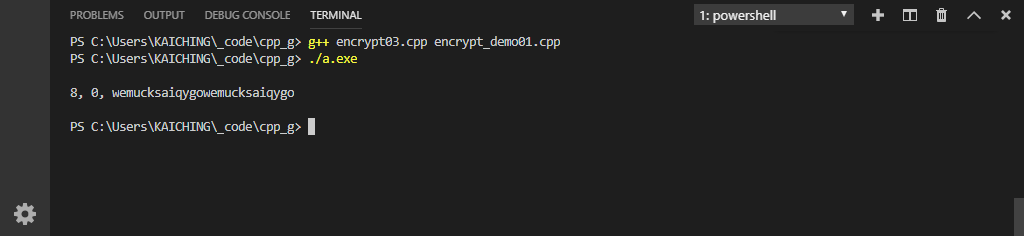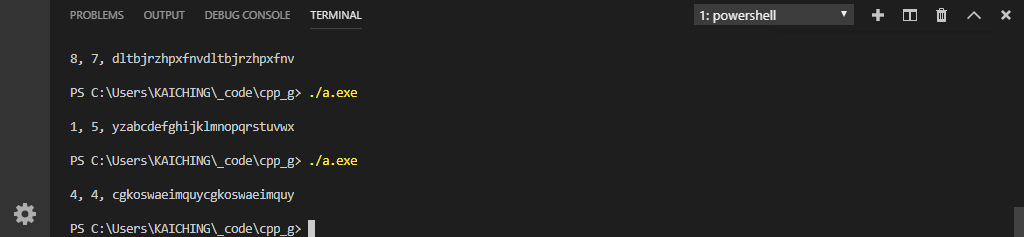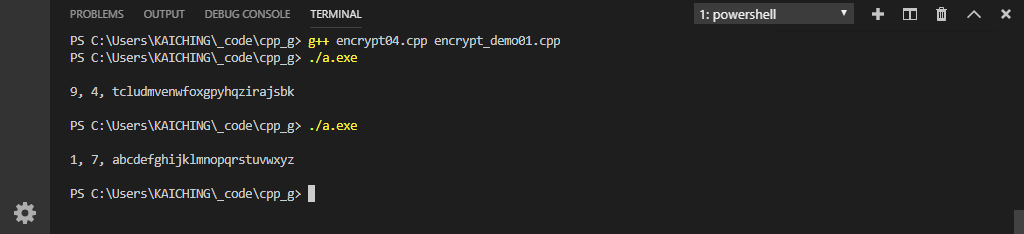# 單元 17 - 修正後的數學公式

Syntax Errors
→ compiler or interpreter
Runtime Errors
→ exception handling
Semantic Errors
→ programmer

y = a * x + b
m = y % n
r = m + diff

``````// 引入標準程式庫中的 cstdlib 及 ctime
#include <cstdlib> // srand(), rand()
#include <ctime>   // time()
// 引入標準程式庫中的 iostream
#include <iostream> // cout, endl

// 引入 Encrypt 類別的標頭檔
#include "encrypt01.h"

// 使用 std 中的兩個名稱
using std::cout; // 標準輸出串流的物件
using std::endl; // 新行符號，等於 '\n'

// Encrypt 的建構函數
Encrypt::Encrypt() {
// 呼叫 setter 設定 code_array
set_code_array();
}

// 設定 code_array 的 setter 成員函數
void Encrypt::set_code_array() {
// 初始化擬隨機數產生器
srand(time(0));
// 取得 a 、 b 值
int a = rand() % 10;
cout << a << ", "; // 印出 a 的值
int b = rand() % 10;
cout << b << ", "; // 印出 b 的值

// 利用公式建立密碼表字串
int x, y, m;
char c = 'a';
string s;
int i;
for (i = 0; i < 26; i++) {
x = c;
y = x * a + b;
m = y % 26;
s += m + 97;
c++;
}

// 將建立好的密碼表直接設定給成員變數
code_array = s;
}

// 回傳密碼表字串的 getter 成員函數
string Encrypt::get_code_array() {
return code_array;
}

/* 程式語言教學誌》的範例程式
http://kaiching.org/
檔名：encrypt03.cpp
功能：實作發展中版本的 Encrypt 類別
作者：張凱慶 */``````if (a % 2) != 0 {
y = a * x + b
m = y % n
r = m + diff
}

``````// 引入標準程式庫中的 cstdlib 及 ctime
#include <cstdlib> // srand(), rand()
#include <ctime>   // time()
// 引入標準程式庫中的 iostream
#include <iostream> // cout, endl

// 引入 Encrypt 類別的標頭檔
#include "encrypt01.h"

// 使用 std 中的兩個名稱
using std::cout; // 標準輸出串流的物件
using std::endl; // 新行符號，等於 '\n'

// Encrypt 的建構函數
Encrypt::Encrypt() {
// 呼叫 setter 設定 code_array
set_code_array();
}

// 設定 code_array 的 setter 成員函數
void Encrypt::set_code_array() {
// 初始化擬隨機數產生器
srand(time(0));

// 取得 a 、 b 值，其中 a 不等於 0 或偶數
int a = 0;
int b = 0;
while (a % 2 == 0) {
// rand() 回傳 0 到 RAND_MAX 之間的擬隨機整數
a = rand() % 10;
b = rand() % 10;
}

// 印出 a 、 b 值
cout << a << ", ";
cout << b << ", ";

// 利用公式建立密碼表字串
int x, y, m;
char c = 'a';
string s;
int i;
for (i = 0; i < 26; i++) {
x = c;
y = x * a + b;
m = y % 26;
s += m + 97;
c++;
}

// 將建立好的密碼表直接設定給成員變數
code_array = s;
}

// 回傳密碼表字串的 getter 成員函數
string Encrypt::get_code_array() {
return code_array;
}

/* 《程式語言教學誌》的範例程式
http://kaiching.org/
檔名：encrypt04.cpp
功能：實作發展中版本的 Encrypt 類別
作者：張凱慶 */``````1. 程式中可能會發生語法錯誤、執行期間錯誤或語意錯誤，編譯器會直接挑出語法錯誤，而執行期間錯誤可用例外處理機制來防範。
2. 發生語意錯誤的程式可順利執行跑出結果，可是結果不符合預期。
3. Encrypt 的數學公式經過再三的測試檢驗，發現問題出在 a0 或偶數的情況，因此要避免 a0 或偶數。

1. 什麼是語法錯誤？試舉出語法錯誤的五個例子。
2. 什麼是執行期間錯誤？想一想有哪些情況會發生執行期間錯誤？又要怎麼樣防範呢？
3. 為什麼語意錯誤最麻煩呢？
4. 我們是用什麼方式找出數學公式的錯誤呢？

1. 承接上一個單元的猜數字遊戲，將新程式寫在 exercise1701.cpp 中，在遊戲迴圈中用變數 times 計算使用者猜測的次數，並在遊戲結束時印出 times
2. 承上題，將新程式寫在 exercise1702.cpp 中，試著加入一個整數參數 lengthArrayNumber() 裡， length 取代固定的數字 4
3. 承上題，將新程式寫在 exercise1703.cpp 中，將原本的數字 1000 改成用標準程式庫 cmathpow() 計算。